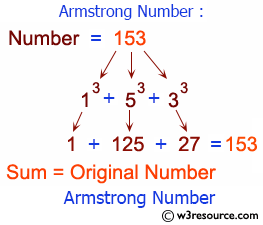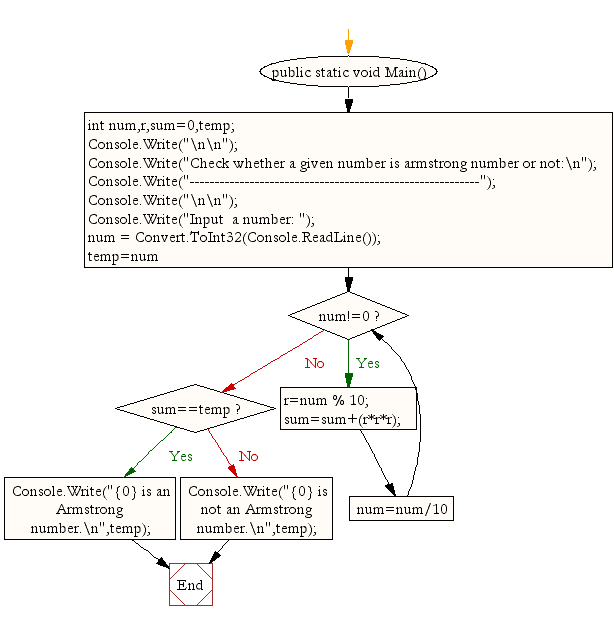﻿ C Sharp - Check if a number is an Armstrong number or not# C# Sharp Exercises: Check whether a given number is an Armstrong number or not

## C# Sharp For Loop: Exercise-29 with Solution

Write a C# Sharp Program to check whether a given number is an Armstrong number or not.Sample Solution:-

C# Sharp Code:

``````/*When the sum of the cube of the individual digits of a number*/
/*is equal to that number, the number is called Armstrong number. For example 153.*/
/*Sum of its divisor is 13 + 53;+ 33; = 1+125+27 = 153*/
using System;
public class Exercise29
{
public static void Main()
{
int num,r,sum=0,temp;

Console.Write("\n\n");
Console.Write("Check whether a given number is armstrong number or not:\n");
Console.Write("----------------------------------------------------------");
Console.Write("\n\n");

Console.Write("Input  a number: ");

for(temp=num;num!=0;num=num/10){
r=num % 10;
sum=sum+(r*r*r);
}
if(sum==temp)
Console.Write("{0} is an Armstrong number.\n",temp);
else
Console.Write("{0} is not an Armstrong number.\n",temp);
}
}
```
```

Sample Output:

```Check whether a given number is armstrong number or not:
----------------------------------------------------------
Input  a number: 153
153 is an Armstrong number.
```

Flowchart:C# Sharp Code Editor:

Contribute your code and comments through Disqus.

What is the difficulty level of this exercise?

Test your Programming skills with w3resource's quiz.

﻿